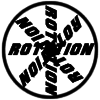#### You may also like### 8 Methods for Three by One

This problem in geometry has been solved in no less than EIGHT ways by a pair of students. How would you solve it? How many of their solutions can you follow? How are they the same or different? Which do you like best?### Rots and Refs

Follow hints using a little coordinate geometry, plane geometry and trig to see how matrices are used to work on transformations of the plane.### Reflect Again

Follow hints to investigate the matrix which gives a reflection of the plane in the line y=tanx. Show that the combination of two reflections in intersecting lines is a rotation.

# The Matrix

##### Age 16 to 18Challenge Level
The point $P=(x,y)$ has image $P'=(ax+by, cx+dy)$. Draw diagrams and consider the effect of the transformation on the line $OP$ joining $P$ to the origin.